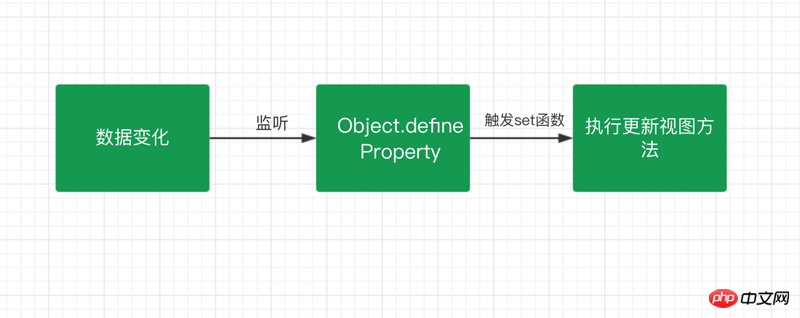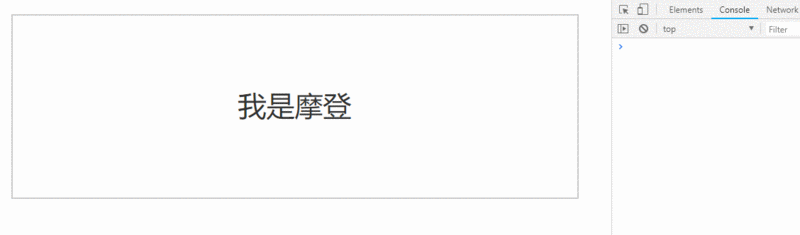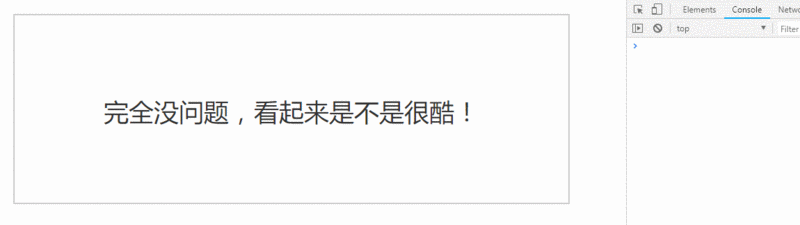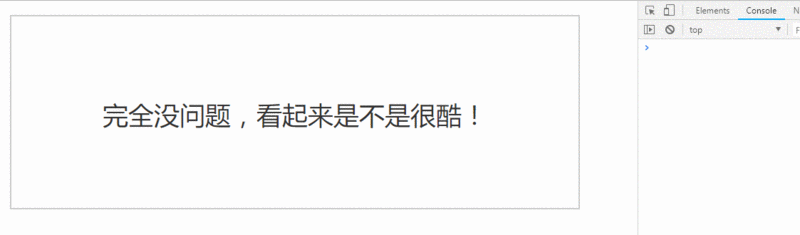# 带你详细实现vue双向绑定

```//代码：
div id= app
input v-model= name type= text
h1 {{name}} /h1
/div
script src= ./js/observer.js /script
script src= ./js/watcher.js /script
script src= ./js/compile.js /script
script src= ./js/index.js /script
script
const vm = new Mvue({
el: #app ,
data: {
name: 我是摩登
/script ```

Angular 的脏查机制

Object.defineProperty

```var people = {
name: Modeng ,
age: 18
people.age; //18
people.age = 20;```

```var modeng = {}
var age;
Object.defineProperty(modeng, age , {
get: function () {
console.log( 获取年龄
return age;
set: function (newVal) {
console.log( 设置年龄
age = newVal;
modeng.age = 18;
console.log(modeng.age);```Observer 监听器：用来监听属性的变化通知订阅者

Watcher 订阅者：收到属性的变化，然后更新视图

Compile 解析器：解析指令，初始化模版，绑定订阅者```function defineReactive(data, key, html' target='_blank'>value) {
//递归调用，监听所有属性
observer(value);
var dep = new Dep();
Object.defineProperty(data, key, {
get: function () {
if (Dep.target) {
return value;
set: function (newVal) {
if (value !== newVal) {
value = newVal;
dep.notify(); //通知订阅器
function observer(data) {
if (!data || typeof data !== object ) {
return;
Object.keys(data).forEach(key = {
defineReactive(data, key, data[key]);
function Dep() {
this.subs = [];
this.subs.push(sub);
Dep.prototype.notify = function () {
console.log( 属性变化通知 Watcher 执行更新视图函数
this.subs.forEach(sub = {
sub.update();
Dep.target = null;```

```var modeng = {
age: 18
observer(modeng);
modeng.age = 20;```

Watcher 主要是接受属性变化的通知，然后去执行更新函数去更新视图，所以我们做的主要是有两步：

```function Watcher(vm, prop, callback) {
this.vm = vm;
this.prop = prop;
this.callback = callback;
this.value = this.get();
Watcher.prototype = {
update: function () {
const value = this.vm.\$data[this.prop];
const oldVal = this.value;
if (value !== oldVal) {
this.value = value;
this.callback(value);
get: function () {
Dep.target = this; //储存订阅器
const value = this.vm.\$data[this.prop]; //因为属性被监听，这一步会执行监听器里的 get方法
Dep.target = null;
return value;
}```

```function Mvue(options, prop) {
this.\$options = options;
this.\$data = options.data;
this.\$prop = prop;
this.\$el = document.querySelector(options.el);
this.init();
Mvue.prototype.init = function () {
observer(this.\$data);
this.\$el.textContent = this.\$data[this.\$prop];
new Watcher(this, this.\$prop, value = {
this.\$el.textContent = value;
}```

``` p id= app {{name}} /p
const vm = new Mvue({
el: #app ,
data: {
name: 我是摩登
}, name ```Compile 解析器

Compile 的主要作用一个是用来解析指令初始化模板，一个是用来添加添加订阅者，绑定更新函数。

```function Compile(vm) {
this.vm = vm;
this.el = vm.\$el;
this.fragment = null;
this.init();
Compile.prototype = {
init: function () {
this.fragment = this.nodeFragment(this.el);
nodeFragment: function (el) {
const fragment = document.createDocumentFragment();
let child = el.firstChild;
//将子节点，全部移动文档片段里
while (child) {
fragment.appendChild(child);
child = el.firstChild;
return fragment;
}```

```Compile.prototype = {
compileNode: function (fragment) {
let childNodes = fragment.childNodes;
[...childNodes].forEach(node = {
let reg = /\{\{(.*)\}\}/;
let text = node.textContent;
if (this.isElementNode(node)) {
this.compile(node); //渲染指令模板
} else if (this.isTextNode(node) reg.test(text)) {
let prop = RegExp.\$1;
this.compileText(node, prop); //渲染{{}} 模板
//递归编译子节点
if (node.childNodes node.childNodes.length) {
this.compileNode(node);
compile: function (node) {
let nodeAttrs = node.attributes;
[...nodeAttrs].forEach(attr = {
let name = attr.name;
if (this.isDirective(name)) {
let value = attr.value;
if (name === v-model ) {
this.compileModel(node, value);
node.removeAttribute(name);
//省略。。。
}```

```function Mvue(options) {
this.\$options = options;
this.\$data = options.data;
this.\$el = document.querySelector(options.el);
this.init();
Mvue.prototype.init = function () {
observer(this.\$data);
new Compile(this);
}```

``` p id= app
h1 {{name}} /h1
script src= ./js/observer.js /script
script src= ./js/watcher.js /script
script src= ./js/compile.js /script
script src= ./js/index.js /script
script
const vm = new Mvue({
el: #app ,
data: {
name: 完全没问题，看起来是不是很酷！
/script ``````function Mvue(options) {
this.\$options = options;
this.\$data = options.data;
this.\$el = document.querySelector(options.el);
//数据代理
Object.keys(this.\$data).forEach(key = {
this.proxyData(key);
this.init();
Mvue.prototype.init = function () {
observer(this.\$data);
new Compile(this);
Mvue.prototype.proxyData = function (key) {
Object.defineProperty(this, key, {
get: function () {
return this.\$data[key]
set: function (value) {
this.\$data[key] = value;
}```